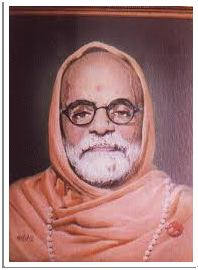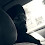## Pages

### Vedic Maths

This series will cover various topics/techniques covered under Vedic Maths. Vedas are very old scriptures of Hinduism. Mathematical methods in Vedas were lost in time, they were rediscovered by Bharati Krishna Tirthaji.Bharati Krishna Tirthaji
Vedic Maths includes various methods/concepts that make mathematical calculations simple. When I was a kid my Dad had presented me a book written by Bharati Krishna Tirthaji. I could not understand much then but my curiosity remained. Recently I bumped into book "Power of Vedic Maths" by Atul Gupta. Author has gone through various methods of Vedic Maths in comprehensive and illustrious way. I would highly recommend this book. My idea here is to explore and explain each method in detail and verify it mathematically. I would not try to explain the Vedic Maths sutra's and their translation, which I leave it to readers.I would be more than happy to hear your feedback.

Following books are highly recommended
1. Vedic Maths by Atul Gupta
2. How to become a Human calculator by Aditi Singhal

Happy Learning
~harish sk

List of articles are

02: Multiplication by 999.... [with same number of digits]
03: Multiplication by 999.... [with greater number of digits]
04: Multiplication by 999.... [with lesser number of digits]
05: Navashesha - remainder of 9
* 06: Navashesha - verification of product of two number
* 07: Navashesha - verification of sum of two number
* 08: Navashesha - verification of subtraction of two number
* 09: Navashesha - verification of square of two number
* 10: Quotient of division by 9 (Method 1)
* 11: Quotient of division by 9 (Method 2)
* 12: Multiplication by 11
* 13: Divisibility test by 11
* 14: Multiplication by 111
* 15: Nikhilam - Multiplication of numbers less than 100
* 16: Nikhilam - Multiplication of numbers above than 100
* 17: Nikhilam - Multiplication of numbers one above 100 and one below 100
* 18: Nikhilam - Multiplication of numbers with secondary base 50 and primary base 10
* 19: Nikhilam - Multiplication of numbers with secondary base 50 and primary base 100
* 20: Nikhilam - Multiplication of numbers with secondary base 500 and primary base 100
* 21: Nikhilam - Multiplication of numbers with secondary base 500 and primary base 1000
* 22: Nikhilam - Multiplication of numbers with secondary base 40 and primary base 10
* 23: Nikhilam - Multiplication of numbers with secondary base 60 and primary base 10
* 24: Nikhilam - Multiplication of numbers with secondary base 300 and primary base 100
* 25: Urdhva Tiryak - Multiplication of two 2 digit numbers
* 26: Urdhva Tiryak - Multiplication of two 3 digit numbers
* 27: Urdhva Tiryak - Multiplication of 3 digit and 2 digit number
* 28: Urdhva Tiryak - Multiplication of two 4 digit number
* 29: Division
* 30: Square of number ending with 5
* 31: Product of number starting with same digit and sum of decimal place is 10
* 32: Dwanda - Introduction
* 33: Dwanda - Square of 2 digit number
* 34: Dwanda - Square of 3 digit number
* 35: Dwanda - Square of 4 digit number
* 36: Dwanda - Square of 5 digit number

#### 1 comment :

1.With Vedic Maths, one can do wonders. :)